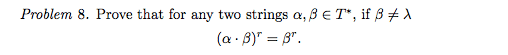# Proof by Induction of shortest suffix of concatenated string

• Enharmonics
In summary, the conversation discusses the use of strings and operators to prove a proposition about concatenation. The base case of the proof is shown and the inductive step is attempted, but it is difficult to prove that a certain string has a specific length. It is suggested to try constructing a new string to continue the proof.

## Homework StatementWherein α, β are strings, λ = ∅ = empty string, βr is the shortest suffix of the string β, βl is the longest prefix of the string β, and T* is the set of all strings in the Alphabet T, |α| denotes the length of a string α, and the operator ⋅ (dot) denotes concatenation of two strings.

## Homework Equations

Proposition 1: ∀α ∈ T* (λ ⋅ α) = α

CONCATENATION, defined recursively:

C-RC0: if |β| = 0, α⋅β = α ⋅ λ = α

C-RC1: if |β| = 1, α⋅β : |α| + 1 → T such that
(α⋅β)(i) = α(i) if i < |α|
(α⋅β)(i) = β(0) if i = |α|

C-RC2: if |β| > 1, α⋅β = (α ⋅ βl ⋅ βr)

## The Attempt at a Solution

I'm using induction on |α| (it seemed like it would be a bit easier this way when I started). I managed to prove the base case:

BASE CASE: Let |α| = 0. Then α = λ and

LHS = (α⋅β)r = (λ⋅β)r = βr = RHS

The inductive step is proving much more difficult, though:

INDUCTIVE HYPOTHESIS: If |α| = n, then (α⋅β)r = βr

LHS = (α⋅β)r = (αl ⋅ (αr ⋅ β))r = (αr⋅β)r = (αr⋅βl⋅βr)r

While I was applying my inductive hypothesis in the step

l⋅(αr⋅β))r = (αr⋅β)r = (αr⋅βl⋅βr)r

I noticed that if I could apply my inductive hypothesis again to the result:

r⋅βl⋅βr)r

This would leave me with the RHS, βr. However, in order to do that I'd have to be able to prove that the string

r⋅βl)

has length n. That's where I'm stuck. I can't think of a way to do that. I tried induction on |β| instead (just to see what would happen) and I ended up getting stuck in a similar way.

Is there a way to prove that |(αr⋅βl)| = n? Or is there a better way to prove this that I'm not seeing? I don't know what else to try at this point. Note that I'm not limited to using only the equations/propositions specified in the homework template. Those are just some easy axioms that were proven in class. I can use any proposition/theorem as long as I show its proof.

#### Attachments

It seems to me that given ##(\alpha_n \cdot \beta)^r = \beta^r##, you need to show that ##(\alpha_{n+1} \cdot \beta)^r = \beta^r##. How would you construct ##\alpha_{n+1}## given ##\alpha_n##?